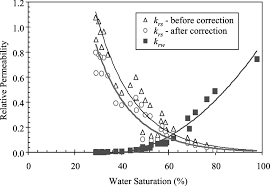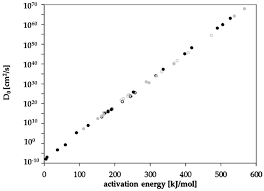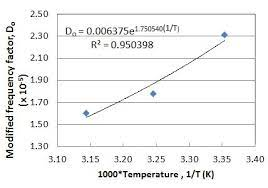## How to Calculate and Solve for Permeability | Mass TransferThe image above represents permeability.

To compute for permeability, five essential parameters are needed and these parameters are Constant (A), Partial Pressure (Po), Activation due to Permeation (Qp), Gas Constant (R) and Temperature (T).

The formula for calculating permeability:

P = APo0.5 . e-Qp/RT

Where:

P = Permeability
A = Constant
Po = Partial Pressure
Qp = Activation due to Permeation
R = Gas Constant
T = Temperature

Let’s solve an example;
Find the permeability when the constant is 12, the partial pressure is 22, the activation due to permeation is 10, the gas constant is 14 and the temperature is 2.

This implies that;

A = Constant = 12
Po = Partial Pressure = 22
Qp = Activation due to Permeation = 10
R = Gas Constant = 14
T = Temperature = 2

P = APo0.5 . e-Qp/RT
P = 12(22)0.5 . e-(10)/(14)(2)
P = 12(4.69) . e-10/28
P = 56.28 . e-0.357
P = 56.28 (0.699)
P = 39.38

Therefore, the permeability is 39.38.

## How to Calculate and Solve for Activation Energy of Diffusion | Mass TransferThe image above represents activation energy of diffusion.

To compute for activation energy of diffusion, four essential parameters are needed and these parameters are Gas Constant (R), Melting Temperature of Metal (Tm), Constant that depends on Metal Crystal Structure (Ko) and Normal Valence in Metal (v).

The formula for calculating activation energy of diffusion:

Q = RTm(Ko + v)

Where:

Q = Activation Energy of Diffusion
R = Gas Constant
Tm = Melting Temperature of Metal
Ko = Constant that depends on Metal Crystal Structure
v = Normal Valence in Metal

Let’s solve an example;
Find the activation energy of diffusion when the gas constant is 24, the melting temperature of metal is 4, the constant that depends on metal crystal structure is 8 and the normal valence in metal is 2.

This implies that;

R = Gas Constant = 24
Tm = Melting Temperature of Metal = 4
Ko = Constant that depends on Metal Crystal Structure = 8
v = Normal Valence in Metal = 2

Q = RTm(Ko + v)
Q = 24(4)(8 + 2)
Q = 24(4)(10)
Q = 960

Therefore, the activation energy of diffusion is 960 J.

Calculating the Gas Constant when the Activation Energy of Diffusion, the Melting Temperature of Metal, the Constant that depends on Metal Crystal Structure and the Normal Valence in Metal is Given.

R = Q / Tm (Ko + v)

Where;

R = Gas Constant
Q = Activation Energy of Diffusion
Tm = Melting Temperature of Metal
Ko = Constant that depends on Metal Crystal Structure
v = Normal Valence in Metal

Let’s solve an example;
Given that the activation energy of diffusion is 9, the melting temperature is 5, the constant that depends on metal crystal structure is 14 and the normal valence in metal is 7.

This implies that;

Q = Activation Energy of Diffusion = 9
Tm = Melting Temperature of Metal = 5
Ko = Constant that depends on Metal Crystal Structure = 14
v = Normal Valence in Metal = 7

R = Q / Tm (Ko + v)
R = 9 / 5 (14 + 7)
R = 9 / 5 (21)
R = 9 / 105
R = 0.085

Therefore, the gas constant is 0.085.
Continue reading How to Calculate and Solve for Activation Energy of Diffusion | Mass Transfer

## How to Calculate and Solve for Frequency Factor of Diffusion | Mass TransferThe image above represents frequency factor of diffusion.

To compute for frequency factor of diffusion, five essential parameters are needed and these parameters are Interatomic Distance (σ), Jump Frequency (vo), Coordination Number (z), Gas Constant (R) and Entropy Change (ΔS).

The image above represents frequency factor of diffusion:

Do = σ²voz/6 . eΔS/R

Where:

Do = Frequency Factor of Diffusion
σ = Interatomic Distance
vo = Jump Frequency
z = Coordination Number
R = Gas Constant
ΔS = Entropy Change

Let’s solve an example;
Find the frequency factor of diffusion when the interatomic distance is 11, the jump frequency is 22, the coordination number is 14, the gas constant is 17 and the entropy change is 10.

This implies that;

σ = Interatomic Distance = 11
vo = Jump Frequency = 22
z = Coordination Number = 14
R = Gas Constant = 17
ΔS = Entropy Change = 10

Do = σ²voz/6 . eΔS/R
Do = (11)²(22)(14)/6 . e10/17
Do = (121)(22)(14)/6 . e0.588
Do = 37268/6 . 1.80
Do = 6211.33 . 1.80
Do = 11185.41

Therefore, the frequency factor of diffusion is 11185.41.